CAT  >  Introduction & Examples: Binary Logic

# Introduction & Examples: Binary Logic - Logical Reasoning (LR) and Data Interpretation (DI) - CAT

 Table of contentsWhat is Binary Logic?Concepts Required to Solve Binary Logic ProblemsBinary Logic Question TypesStep Wise Solved ExamplesThe logical Reasoning section in the Common Admission Test (CAT) carries a couple of questions on binary logic. The application of this logic is more in finding out the truthfulness of the statements made.
Each question contains two logic streams that have to be matched, in order to get the correct answer.

## What is Binary Logic?

The Binary logic derives its name from the Boolean logic which is the synonym to two-value logic system. In fact, it is the sub area of algebra called Boolean algebra in which the values of the variables are the truth values - true and false.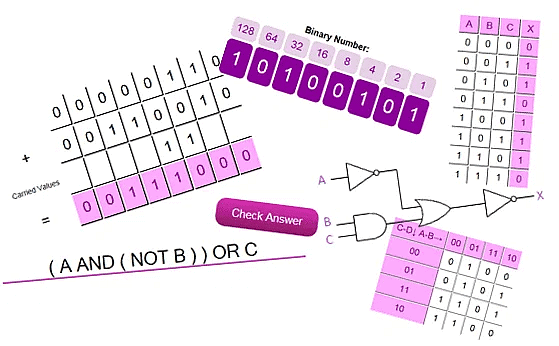• Binary logic consists of a set of rules based on operations of mathematical binary operations of NOT, AND, and OR.
• There are only two logical propositions either True or False. Based on the given instructions the possibilities should be made for the given problem.
• Notations vary greatly, so it is important to remain consistent in how one writes out binary logic.

## Concepts Required to Solve Binary Logic Problems

In these types of problems: we have people who either speak a true statement or a false statement. Hence these people are divided into three categories:

1. TruthTeller: This person will always speak the truth. All the statements made by this person are true. 2. Liar: This person will always tell a lie. All statements made by this person are false.

3. Alternators: This person always alternates between the truth and the lie.

• If first statement of this person is true, then second will be false, third will be true and so on.
• Similarly, if first statement made by this person is false, then second will be true, third will be false and so on.
• There is no particular number of true statements or false statements made by this person but order is always TRUE-FALSE-TRUE- or FALSE-TRUE-FALSE.

Binary logic questions are all about making some assumptions. These assumptions may give rise to some contradictions which are the indicators that our assumptions are wrong. If for any assumption we do not get even a single contradiction, then that is the solution for the given  problem.

## Binary Logic Question Types

### Type 1

Three persons speak two statements each - one of which is true, the other is false.
The two logic streams to be considered are:

1. The statement logic of the contents is stated within.
2. The ingredients i.e. the fact- one is true other will be naturally false or vice versa.

### Type 2

Two types of persons are found in this type of question.

1. Those who always speak the truth.
2. Those who always tell a lie.

If a person always speaks the truth, then whatever statement he says must be true. However, a clash might be there if it can be seen that the statement is obviously false.

Question for Introduction & Examples: Binary Logic
There are two types of inhabitants in Tatabalery- A type & B type. The A type of inhabitants always speak the truth and the B Type of inhabitants always lie.

Q: Booker says," At least one person among Shane and I always lie." What types are Booker and Shane respectively?

## Step Wise Solved Examples

Example 1: Three boys- Aman, Bagheer, and Chiru replied to the question, “Who among you is a Doctor” in the following manner:
We know exactly one of these boys is a Doctor, one is a Painter and one is an Athlete.
Further, one always speaks the truth, one always lies, and one alternates between the truth and the lie.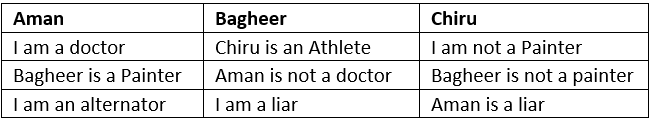### Steps to Solve Binary Logic

With the help of some statements made by these people, we might be able to identify them without any assumption. Here is a list of 4 statements that one must always look out for to make the task easier. If any one of these is made by any person, then we can categorize them as explained below.
1. I am a liar

• Consider if a truth-teller says, “I am a liar”, which is a lie as a truth-teller can only say, “I am a truth-teller”. Hence, we can conclude that the person who said “I am a liar” is not the truth-teller.
• Similarly, if a liar says that he/she is a liar then that statement will be true but the liar will always speak the lie. None of the statements made by him/her can be true. We can conclude this statement cannot be made by a liar.
• The only category of person who can speak this statement can be the alternator. He can alternate between the truth and the lie. Since he/she is not a liar but he/she can still make a false statement, alternator is the only category of people who can make the statement, “I am a liar”. The statement in itself will be a lie.
• This gives us another hint that the statement proceeding and the statement preceding this statement will always be true.

2. I am not a truth-teller

• Similar to the explanation above, a truth-teller can never make this statement because if he/she makes this statement then it will be a lie which contradicts the fact that a truth-teller always speaks the truth.
• If the liar makes the above statement, then it will be the truth for him which again contradicts the fact that a liar will always lie.
• Hence, a liar cannot make this statement.
• The alternator can say, “I am not a truth-teller”, as he can say either the truth or the lie. This statement will be a true statement for him, which gives us another hint that the statements preceding and proceeding this statement are the lie.

3. I am an alternator

• A truth-teller cannot make this statement as this statement will be a lie for him which conflicts the fact of the truth-teller.
• A liar can make this statement as this statement will be a lie for him/her.
• An alternator can also make this statement and this will be the truth for the alternator.
• We can conclude that this statement can be made by the liar or the alternator.

4. I am not an alternator

• Similar to the above statement, a liar will not make this statement.
• A truth-teller can make this statement.
• An alternator can make this statement and this time it will be the lie for him/her.

### Summary to Explain Example 1

• In our example question, Bagheer makes the statement, “I am a liar”, which implies that Bagheer is the alternator. Also, Aman said that he is an alternator, which implies that he can be either a liar or an alternator. Now, since Bagheer is the alternator, Aman is definitely the liar.
• This leaves us with Chiru as the truth-teller. Since Bagheer is the alternator, so the statements proceeding/preceding the statement, “I am a liar” are true, we can conclude that Aman is not a Doctor.
• Also as Chiru is a truth-teller, according to his statements, Bagheer is not a painter and he himself is not a painter, thus Aman is the painter. Now, Bagheer’s first statement is a lie (since third statememt is also a lie, so the order for Bagheer’s statements will be 1st-false,2nd-true,3rd-false), this means Chiru is not an athlete, which leaves us with the only option for Chiru as Doctor and Bagheer will then be an athlete.
• To summarize our findings: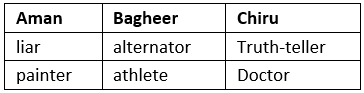So the answer to the question, ‘Who is a Doctor’, is Chiru.
Other statements such as, “I am a truth-teller”, can be made by all the three categories of person and so will not be of much help to us.

Step 2

• In some cases, where none of the four statements as mentioned in step 1 was made by anyone, we will use the assumption-iteration method.
• In this method we will assume the first person as the truth-teller and based on his statements we will try to find conflicts or contradictions that may arise due to the statements made by others.
• We will also use the assumptions-iteration method when statement 3 or 4 mentioned in step 1 is made by anyone as these statements will leave us with two options.

Example 2: Utkarsh, Ravi, and Shivam made the following statements regarding the type of vehicle they own. Each one of them belongs to exactly one category of truth-teller, liar or alternator. Only one among them is a truth-teller. Further, we know each of them own a different vehicle and each of them own exactly one among car, cycle and bike.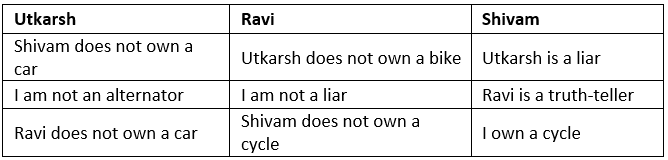Solution:

• Now, Utkarsh says that he is not an alternator and as per step 1 we know that he can be a truth-teller or an alternator.
• To proceed with the approach, we will first assume that Utkarsh is the truth-teller and all the statements are true. We know exactly one among them is a truth –teller and we already assumed Utkarsh as the truth-teller.
• The question does not specifically mention the exact number of each category. From Utkarsh’s statements, we know neither Shivam owns a car nor Ravi. Reading Ravi’s statements, since his last statement is true so he should be an alternator (he can’t be the truth-teller as there is only one truth-teller which we already assumed as Utkarsh).
• This implies his second statement should be false but as per the statement made by Ravi, the second statement is also true.
• This is a contradiction to our assumption which means that our assumption is wrong.
We have to go for the second interation knowing Utkarsh is the alternator.
• As he is not the truth-teller, he has to be an alternator. His second statement is false which automatically makes his first and second statements true.
• We can deduce that Utkarsh owns a car as none of Ravi and Shivam owns a car as per Utkarsh’s statements. Shivam’s first statement is a lie so he can be a liar or an alternator. Since there is one truth-teller and the only option for the truth-teller is Ravi thus we can say Ravi is the truth-teller.
• This makes Shivam’s second statement true and so we know that Shivam is an alternator. Shivam does not own a cycle makes Ravi own a cycle. Shivam owns a Bike.

Directions for examples 3 & 4: Read the following paragraph and answer the questions that follow.
There are two types of inhabitants in Tatabalery- A type & B type. The A type of inhabitants always speak the truth and the B Type of inhabitants always lie.

Example 3: Rocky says, " I always lie." which type of inhabitant is he?

(a) A
(b) B
(c) EitherA or B
(d) The given statement is infeasible

Example 4: Peter says," According to Rocky, I always speak the truth." Which of the following is a correct conclusion?

(a) Peter has to be of type A
(b) Peter has to  be of type B
(c) Rocky has to  be of type A
(d) Rocky has to be of type B

### Summary

• We conclude this post with this example where there are two alternators and one truth-teller.
• The question specifically mentions the number of truth-tellers in order to avoid any ambiguity.
• Always look for Step 1 statements in order to make the task simpler and time-saving and then go for the assumption-iteration method.
The document Introduction & Examples: Binary Logic | Logical Reasoning (LR) and Data Interpretation (DI) - CAT is a part of the CAT Course Logical Reasoning (LR) and Data Interpretation (DI).
All you need of CAT at this link: CAT

## Logical Reasoning (LR) and Data Interpretation (DI)

125 videos|168 docs|117 tests

## FAQs on Introduction & Examples: Binary Logic - Logical Reasoning (LR) and Data Interpretation (DI) - CAT

 1. What is binary logic?Binary logic is a fundamental concept in computer science and mathematics that deals with logical operations on binary variables. It involves using two values, typically represented as 0 and 1, to represent true and false or on and off. Binary logic forms the basis of digital circuits and is used extensively in computer programming and electronic engineering.
 2. What are the concepts required to solve binary logic problems?To solve binary logic problems, you need to understand several key concepts. These include: 1. Boolean algebra: Boolean algebra is a branch of algebra that deals with binary variables and logical operations, such as AND, OR, and NOT. It provides the foundation for binary logic and helps in solving complex logic problems. 2. Truth tables: Truth tables are used to represent the possible combinations of inputs and their corresponding outputs in binary logic. They help in understanding the behavior of logical operations and analyzing the validity of logical expressions. 3. Logic gates: Logic gates are electronic devices that perform basic logical operations, such as AND, OR, and NOT, on binary inputs. They are the building blocks of digital circuits and are used to implement binary logic in hardware. 4. Boolean expressions: Boolean expressions are symbolic representations of logical operations using variables, logical operators, and parentheses. They are used to describe and manipulate binary logic problems. 5. Problem-solving techniques: Solving binary logic problems requires logical reasoning, deduction, and problem-solving skills. Techniques such as using truth tables, simplifying Boolean expressions, and applying logical rules are often employed to find the solution.
 3. What are the different types of binary logic questions?There are several types of binary logic questions that can be encountered in exams or problem-solving scenarios. Some common types include: 1. Truth table problems: These questions involve constructing or analyzing truth tables for given logical expressions or circuits. 2. Logic gate problems: These questions require identifying the type of logic gate(s) needed to implement a given logical operation or circuit. 3. Boolean expression simplification problems: These questions involve simplifying complex Boolean expressions using algebraic laws and logical rules. 4. Logical deduction problems: These questions require deducing the correct answer based on a given set of logical statements or conditions. 5. Circuit design problems: These questions involve designing digital circuits using logic gates to perform specific logical operations.
 4. Can you provide an example of solving a binary logic problem step by step?Certainly! Here's an example of solving a binary logic problem step by step: Problem: Simplify the Boolean expression (A + B) . (A + C) . (B + C). Step 1: Apply the distributive law to expand the expression: (A + B) . (A + C) . (B + C) = (A . A + A . C + B . A + B . C) . (B + C) = (A + A . C + B . A + B . C) . (B + C) Step 2: Simplify the terms using the identity law: (A + A . C) = A (B + B . C) = B The expression becomes: (A + A . C + B . A + B . C) . (B + C) = (A + B . A + A . C + B . C) . (B + C) = (A + A . C + B . C) . (B + C) Step 3: Apply the distributive law again: (A + A . C + B . C) . (B + C) = (A + A . C) . (B + C) + (B . C) . (B + C) = A . (B + C) + C . (B + C) The simplified expression is: A . (B + C) + C . (B + C)
 5. How is binary logic used in computer programming?Binary logic is extensively used in computer programming to make decisions, control the flow of execution, and implement logical operations. Programmers use boolean variables, which can only hold two values (true or false), to represent binary logic in programming languages. Binary logic is used in conditional statements, such as if-else statements and switch statements, to execute different blocks of code based on certain conditions. It is also used in loop structures, such as while loops and for loops, to repeat code execution until a certain condition is met. Logical operators, such as AND, OR, and NOT, are used to combine boolean expressions and perform complex logical operations in programming. These operators help in making comparisons, evaluating conditions, and manipulating boolean values. Overall, binary logic forms the foundation of decision-making and control structures in computer programming, allowing programmers to create efficient and logical algorithms.

## Logical Reasoning (LR) and Data Interpretation (DI)

125 videos|168 docs|117 tests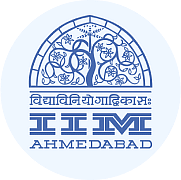Explore Courses for CAT exam### How to Prepare for CAT

Read our guide to prepare for CAT which is created by Toppers & the best Teachers
Signup to see your scores go up within 7 days! Learn & Practice with 1000+ FREE Notes, Videos & Tests.
10M+ students study on EduRev
Track your progress, build streaks, highlight & save important lessons and more!
Related Searches

,

,

,

,

,

,

,

,

,

,

,

,

,

,

,

,

,

,

,

,

,

;